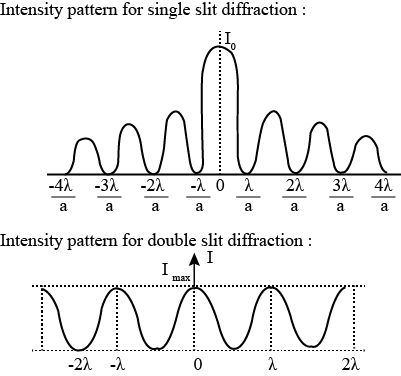Ravela Da Cruz

HS-PS4-3

diffraction of light

single-slit diffraction experiment

single-slit diffraction formula

double-slit experiment

# Single Slit Diffraction Study Guide

The pattern of diffraction of light in the single-slit experiment is different from those formed by double slits.

# INTRODUCTION

When we examine light waves, we learn about their many features like, how they reflect back in the process of reflection, how they change direction in the process of refraction, and how they interfere with other light waves. But, what do you believe happens when light waves collide with a gap? Will it go straight through the gap, or will it shift its direction? Let's investigate.

## DIFFRACTION OF LIGHT AND SINGLE-SLIT DIFFRACTION

• The curving of light waves around a tight turn of an obstacle or an opening is known as the diffraction of light.
• The single slit diffraction’s meaning is that an alternating dark and bright pattern can be seen when light is imposed on a slit with a size corresponding to the wavelength of light.
• When light strikes the gap, secondary wavelets form at each point, as per Huygens' rule.
• These wavelets start out in a phased manner and then disperse on all sides.
• Each one of them covers a specific path to reach any location on the screen.
• Due to the path difference, they reach diverse phases and may interact either constructively or destructively.

## THE SINGLE SLIT DIFFRACTION EXPERIMENT

• The single-slit diffraction experiment is similar to the double-slit performed by Thomas Young in 1801.
• Here, monochromatic light is projected through a slit of finite length.
• The only differences between a single slit and a double-slit experiment are the diffraction patterns and the intensity graphs.Source

The double-slit experiment patterns differ from the single-slit diffraction pattern as the width and intensity reduce as we move away from the central maximum.

## SINGLE SLIT DIFFRACTION FORMULASSource

The path difference between two rays at an angle θ is given as ∆L= a/2 sin θ (valid only when D>> a )

The bright fringes appear at θ → sin ^-1 ( ± 3ℷ/2), sin^-1 (± 5ℷ/2)

The dark fringes appear at a sin θ = mℷ

The angular width of the central maximum is 2θ = 2λ/a

The linear width of central maxima is  2λDa.

Here,

∆L is the path difference

λ is the wavelength of the projected light

D is the distance between a slit and a screen

a is the  slit width

And θ is the angle made with the undeviated direction of light.

# SUMMARY

• Diffraction is the curving of light waves around a tight turn of an obstacle or an opening.
• In a single slit experiment, an alternating dark and bright pattern can be seen when light is imposed on a slit with a size corresponding to the wavelength of light.
• The only differences between a single slit and a double-slit experiment are the diffraction patterns and the intensity graphs.

## FAQs

Q. What is the condition for constructive interference?

For the constructive interference to take place, the phase difference between the two waves must be an even multiple of pie.

Q. What is the condition for destructive interference?

For the destructive interference to take place, the phase difference between the two waves must be an odd multiple of pie.

Q. What is temporal coherence?

The average correlation between the value of a wave and itself delayed by any pair of times is known as temporal coherence.

We hope you enjoyed studying this lesson and learned something cool about Single Slit Diffraction! Join our Discord community to get any questions you may have answered and to engage with other students just like you! We promise, it makes studying much more fun!😎

## REFERENCE

1. Single-slit Diffraction: https://www.ck12.org/physics/single-slit-diffraction/lesson/Single-Slit-Interference-PHYS/?referrer=concept_details Accessed 14th April 2022.
2. Diffraction: https://en.wikipedia.org/wiki/Diffraction Accessed 14th April 2022.